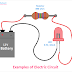## Electric Circuit Definition

Electric Circuit is a closed path consisting of different types of electrical active or passive components where the electric current can easily flow from the power source to load. When we talk about the generation of electricity, consumption of electricity, the concept of the electrical circuit must come. An electrical circuit helps to interconnect the different electrical and electronic components and provides a conductive medium to flow the electric current. All the power sources, loads, and components are connected with an electrical wire.

## Electric Circuit Examples

An LED connected with a battery in series with a resistor can be an example of electric circuit.

Here, the battery is the power source that provides the Direct Current(DC) or DC voltage. The LED is the load that consumes electrical energy. The resistor is the circuit component. It is a passive component. The main function of the resistor is to oppose the electric current. In this circuit, the resistor limits the excess current to flow to the LED more than its rated current. Also, the resistor makes a voltage drop to apply the exact voltage across the LED as its rating. If excessive voltage is applied to the LED, it will be damaged.

## Electric Circuit Components and Parts

The main parts of an electric circuit are the power source, load, and conducting medium. This also can be as the components of an electric circuit. The resistor, capacitors, inductors, diodes, transistors are examples of electric circuit components. There are mainly two types of electric circuit components - 1. Active Components and 2. Passive Components

Active components are those that need an external voltage or biasing for their operation. For example, a diode will conduct current when it is connected in a forward bias. On the other hand, passive components are those that do not require any external power supply or biasing for their operation. For example, a resistor can work in any direction or any voltage and current.

## Electric Circuit Types

There are various types of electric circuits such as - 1. Open Circuit 2. Closed Circuit  3. Series Circuit  4. Parallel Circuit

### Open Circuit

As its name suggests the circuit is not closed or the electric current does not get any closed path for flowing. So in an open circuit electric current never flow. In practice, the open circuit does not do any useful work, there is no power consumption and no power loss.

### Closed Circuit

Closed-circuit is that where the electric current got a closed path to flow from the source to load. Here, the electrical power transformation and consumption both are happens. In general, all the live electric circuits are examples of closed circuits.

### Series Circuit

Series circuit is where all the electrical components and loads are connected in series. In a series electrical circuit, current flows through all the loads are equal or identical but the voltage across all the loads is different. The voltage across each load depends upon the current flows through them and their internal resistance.

### Parallel Circuit

The parallel circuit is where all the electrical components or loads are connected in parallel. Here, the current flows through each load are different but the voltage across all loads is constant. Here the current flows through each load depending upon the applied voltage across the load and their internal resistance.

What is Electric Circuit? Examples, Types, ComponentsReviewed by Author on January 23, 2022 Rating: 5